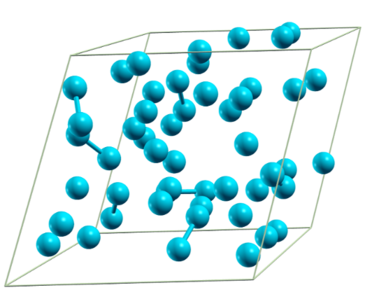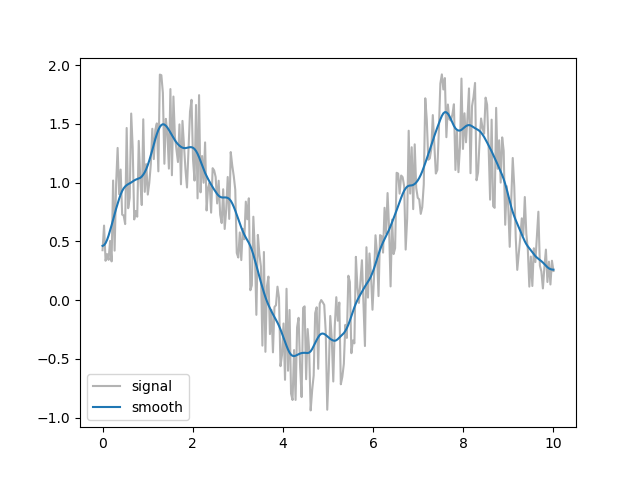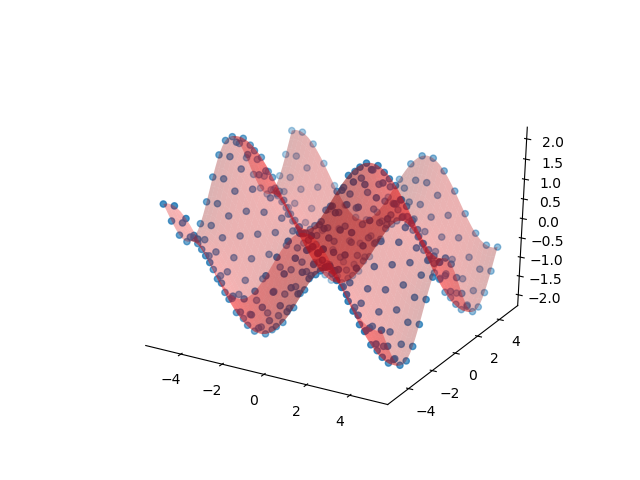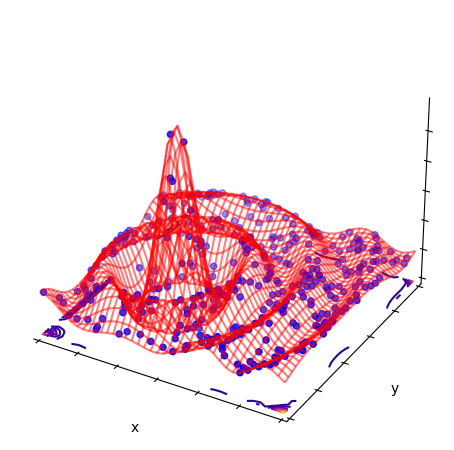# Tutorial¶

Here we collect random short code snippets showing how to perform various common tasks.

## Using ASE?¶

No problem, use atoms2struct() and struct2atoms() to convert back and forth. If you have a Structure, you can also use the get_ase_atoms() method, which is the same as struct2atoms(struct).

For basic ASE compatibility, you may get away with get_fake_ase_atoms(). That creates an object which behaves like ase.Atoms without the need to have ASE installed.

## Parse SCF, relax or MD output¶

Parse SCF run from QE (single Structure):

>>> from pwtools import io
>>> st = io.read_pw_scf('pw.scf.out')
>>> # natoms=2
>>> st.coords_frac
array([[0.  , 0.  , 0.  ],
[0.25, 0.25, 0.25]])
>>> st.etot
-258.58148870118305
>>> st.stress
array([[9.825, 0.   , 0.   ],
[0.   , 9.825, 0.   ],
[0.   , 0.   , 9.825]])


Variable cell relax run from QE into Trajectory. Use the MD parser:

>>> tr = io.read_pw_md('pw.vc_relax.out')
>>> tr.nstep
7
>>> tr.etot - tr.etot[-1]
array([-0.74763576,  0.68611164,  0.22244653, -0.06793023, -0.01616479,
0.00121567,  0.        ])
>>> tr.volume
array([54.12697068, 51.59033133, 49.81153398, 50.1512203 , 50.26524368,
50.25804864, 50.25804864])
>>> # [trace(s)/3 for s in tr.stress]
>>> tr.pressure
array([ 2.60203333e+01, -5.28800000e+00, -2.22400000e+00,  6.80000000e-01,
1.14000000e-01, -6.30000000e-02, -2.33333333e-02])
>>> tr.cryst_const[-1,...]
array([  3.29944506,   3.29944506,   5.33081055,  90.        ,
90.        , 120.00001397])
>>> # nstep=7, natoms=4
>>> tr.coords.shape
(7, 4, 3)


Now an MD from CP2K:

>>> tr = io.read_cp2k_md('cp2k.out')
>>> tr.nstep
123456
>>> # extract first and last Structure objects
>>> st_first = tr
>>> st_last = tr[-1]
>>> # slice out a part of the trajectory
>>> tr_middle = tr[100:500]
>>> # use every 5th step
>>> tr[::5]


## Binary IO¶

You can save a Structure or Trajectory object as binary file:

>>> # save to binary pickle file
>>> tr.dump('traj.pk')


and read it back in later using read_pickle()

>>> tr = io.read_pickle('traj.pk')


which is usually very fast.

## View a structure or trajectory¶

Supported viewers are xcrysden, jmol, avogadro and VMD. Look at single structs or trajectories.

>>> from pwtools import visualize, random
>>> st = random.random_struct(['Si']*50)
>>> visualize.view_xcrysden(st)
>>> ##visualize.view_jmol(st)Andf you have VMD installed, view a random MD trajectory:

>>> import os
>>> from pwtools import crys
>>> tr = crys.concatenate([random.random_struct(['Si']*10) for i in \
...                        range(5)])
>>> # vmd startup script
>>> vmd_script = os.path.dirname(visualize.__file__) + \
'/examples/vmd/nice_bonds.tcl'

>>> visualize.view_vmd_axsf(tr, options='-e %s' %vmd_script)


## Find Monkhorst-Pack k-grid sampling for a given unit cell¶

Say you know from a previous convergence study that a k-grid spacing of h=0.5 1/Angstrom is OK. Now you have a slab or other super cell of your structure and you want to know “what k-grid do I need to get the same accuracy”. Simple:

>>> from pwtools import crys
>>> # new cell in Angstrom
>>> cell=np.diag([8,8,5])
>>> crys.kgrid(cell, h=0.5)
array([2, 2, 3])


OK, so use a $$2\times2\times3$$ MP grid. Instead of defining cell by hand, you could also build your structure, have it in a Structure object, say st and use st.cell instead.

## Find spacegroup¶

Say you have a Trajectory tr, which is the result of a relax calculation and you want to know the space group of the final optimized structure, namely tr[-1]:

>>> from pwtools import symmetry, crys
>>> symmetry.get_spglib_spacegroup(tr[-1], symprec=1e-2)


Or build one using ASE:

>>> import ase.build
>>> at = ase.build.bulk(name='AlN', crystalstructure='rocksalt', a=3)
>>> symmetry.spglib_get_spacegroup(crys.atoms2struct(at))
(225, 'Fm-3m')


## Smoothing a signal or a Trajectory¶

Smoothing a signal (usually called “time series”) can be done by convolution with another function (e.g. with scipy.signal.convolve or scipy.signal.fftconvolve). We implement advanced methods which add edge effect handling: pwtools.signal.smooth(). The same can be applied to a Trajectory, which is just a “time series” of Structures. See pwtools.crys.smooth():

>>> a = rand(10000)
>>> a_smooth = signal.smooth(a, scipy.signal.hann(151))
>>> tr = Trajectory(...)
>>> tr_smooth = crys.smooth(tr, scipy.signal.hann(151))


Example:

>>> from pwtools.signal import smooth
>>> from pwtools import mpl
>>> from scipy.signal import hann
>>> fig,ax = mpl.fig_ax()
>>> x = np.linspace(0,10,300)
>>> y = np.sin(x) + np.random.rand(len(x))
>>> k = hann(21)
>>> ax.plot(x, y, color='0.7', label='signal')
>>> ax.plot(x, smooth(y, k), label='smooth')
>>> ax.legend()
>>> mpl.plt.show()The same is applied to each atomic coordinate in pwtools.crys.smooth().

Find more about edge effects in examples/lorentz.py and the doc string of pwtools.signal.smooth().

## Interpolation and fitting¶

Care for some surface data? Here we fit with a 2D polynomial:

>>> from pwtools import num, rbf, mpl
>>> fig,ax = mpl.fig_ax3d(clean=True)
>>> dd = mpl.get_2d_testdata(20)
>>> ax.scatter(dd.xx, dd.yy, dd.zz)
>>> # same as
>>> #   num.Interpol2D(dd=dd, what='poly', deg=5)
>>> #   num.Interpol2D(dd.XY, dd.zz, what='poly', deg=5)
>>> f = num.PolyFit(dd.XY, dd.zz, deg=5)
>>> ddi = mpl.get_2d_testdata(50)
>>> ddi.update(zz=f(ddi.XY))
>>> ax.plot_surface(ddi.X, ddi.Y, ddi.Z, alpha=0.3, color='r')2D interpolation of samples of a “mexican hat” function $$\sin(r)/r$$ (examples/rbf/surface.py), also using Rbf. See Radial Basis Function interpolation an regression for more. Similar to the PolyFit example above:

>>> # same as
>>> #   num.Interpol2D(dd=dd, what='rbf_multi')
>>> #   num.Interpol2D(dd.XY, dd.zz, what='rbf_multi')
>>> f=rbf.Rbf(dd.XY, dd.zz)## Avoid auto-calculation for big MD data¶

If you have really big MD data (say several GB), then the auto-calculation of missing properties might take long and/or fill up all memory. To avoid that, call the parser explicitly and say auto_calc=False when creating the Trajectory, which will deactivate auto-calculation. It will only do unit conversion to eV, Ang, etc. [you can of course also access the parser’s attributes directly, e.g. pp.coords in the unit of the MD code (e.g. Bohr) instead of tr.coords in Ang].

This is an example for parsing LAMMPS dcd binary data (log.lammps is the logfile and the default binary file is lmp.out.dcd).

>>> pp = parse.LammpsDcdMDOutputFile('log.lammps')
>>> tr = pp.get_traj(auto_calc=False) # default is auto_calc=True


In order to maximally reduce data, you can tell the parser to parse only certain things:

>>> pp.set_attr_lst(['etot', 'coords', 'temperature'])
>>> tr = pp.get_traj(auto_calc=False)


You may also use auto_calc=True here and see what will be auto-calculated from this minimal input data.

Of course you need to know what can be found in the MD data (e.g. if the MD code writes no fractional coords, then parsing coords_frac won’t work).

To find out what can be parsed, also check which get_*() methods the parser implements (mind also base classes, best is to use Tab completion in ipython: >>> pp.get_<tab> or have a look at the API documentation).